## 2.2 Used symbols

To express the relation between one action and another, there exist some international icons. The basic operators you must know are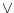,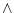,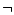,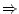. The others are more complex, but here I put all of them as a reference, to be able to find them if you were searching any of them.or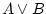is true whenever one of the two, or both, are true.and To make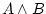true, both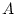and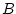have to be true.not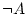only is true whenis false.implies Shows consequence. The expression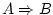says that whenholds, so does. In addition,is considered true except for the casetrue andfalse. To understand that, think of anwhich impliesand ask yourself: is it possible thatis true but not? Anyway, don't worry about that, it's not important right now.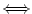if and only if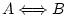is the same as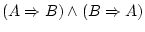. It means that fromwe can deduceand viceversa, so they are equivalent.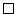false The empty square represents false (the binary 0). Technically, it represents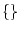.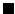true The filled square represents true (the binary 1). Technically, it represents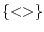.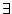exists...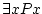can be read there exists an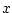such that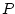of. If in our domain, we can find an element (or more) which makes true the propertyapplied to that element, then the formula is true.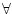for all...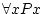can be read for all,of. If all elements we are working with make the propertybecome true, then the formula is true.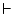thenis the symbol of the sequent, which is the way of saying when all this from the left happens, then it also happens all this from the right''. There exist valid sequents, like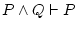or like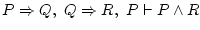. But there are also invalid ones, like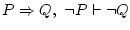. The objective of natural deduction is to prove that a sequent is valid.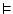valid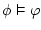means that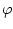is logical consequence of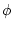, but when one writes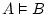, what we mean is that the sequent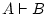is valid, that is, we could somehow prove it, and now is considered true for any interpretation of the predicate symbols.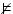invalid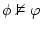means thatis not logical consequence of. If you can find a series of values (model) which maketrue butfalse, then invalidity is proven.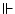satisfiable A set of formulas is satisfiable if there exists a series of values (model) which can make all of them true at the same time.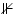unsatisfiable A set of formulas is unsatisfiable if there isn't any combination of variables (model) which can make all of them become true at the same time.

Daniel Clemente Laboreo 2005-05-17